Categories

# Atmospheric Humidity

Because of the high variability of water vapor in air, meteorologists like to use separate equations of state for dry air and water vapor. This is legitimate following Dalton’s law, which states that the total pressure of a mixture of gases is the sum of the partial pressures of its individual components. The equation of state for dry air is given by

pd = ρdRdT (6)

where the specific gas constant for dry air is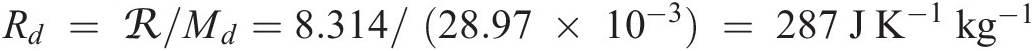. A similar equation can also be applied to water vapor (or any chemical constituent). The partial pressure of water vapor is commonly noted e and the equation of state is expressed by

e = ρwRwT

where ρw is the water vapor mass density [kg m–3] and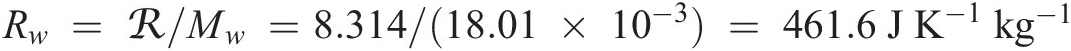is the specific gas constant for water vapor with a molar mass Mw of 18.01 × 10–3 kg mol–1.

Note that the total air pressure is

p = pd + e.

The volume mixing ratio Cw and mass mixing ratio μw of water vapor are expressed by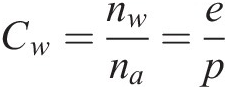and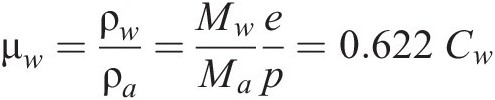where nw and na are the number densities [molecules m–3] of water and moist (total) air, respectively, Mw = 18.01 × 10–3 kg mol–1 is the molar mass of water, and Ma is the molar mass of moist air: Ma = (1 – Cw)Md + CwMw. Meteorologists conventionally call μw the specific humidity (and write it q). They instead define the water vapor mass mixing ratio rw as the ratio between the water vapor density ρw and the dry air density ρd, where

ρd = ρa  ρw: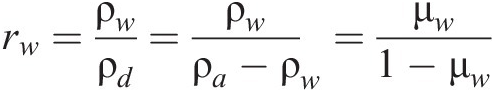The equation of state (6) for dry air can be applied to moist air if the temperature T is replaced by the virtual temperature Tv, the temperature at which dry air has the same pressure and density as moist air. Thus one writes

p = ρaRdTv

From the above equations, it follows that

or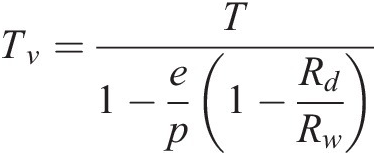with Rd/Rw = Mw/Md = 18/28.97 = 0.621. A good approximation to this expression is provided by

Tv ≈ (1 + 0.61 rw)T

Phase transitions of atmospheric water play a crucial role in meteorology. The relative humidity RH [percent] is expressed by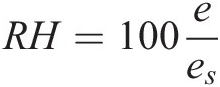where es is the saturation pressure at which water vapor is in equilibrium with the condensed phase (liquid or ice). For a saturated atmosphere (e = es), condensation and evaporation are in balance. One shows easily that the water mass mixing ratio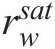corresponding to saturation is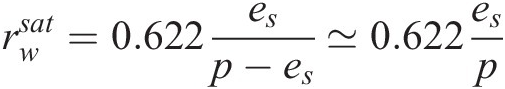Its value is inversely proportional to the total pressure and is a function of temperature because the saturation pressure es varies with temperature (see later). An atmosphere with e < es is called subsaturated while one with e > es is called supersaturated. A supersaturated atmosphere leads to cloud formation, contingent on the presence of suitable aerosol particles to provide pre-existing surfaces for condensation and overcome the energy barrier from surface tension. These particles are called cloud condensation nuclei (CCN) for liquid-water clouds and ice nuclei (IN) for ice clouds. Water-soluble particles greater than 0.1 μm in size are adequate CCN, and are sufficiently plentiful that liquid cloud formation takes place at supersaturations of a fraction of a percent. Ice nuclei are solid particles such as dust that provide templates for ice formation and are present at much lower concentrations than CCN. Because of the paucity of IN, clouds may remain liquid or mixed ice–liquid at temperatures as low as –40 °C; one then refers to the metastable liquid phase as supercooled.Phase equilibrium for water is defined by the phase diagram in Figure 5. Lines on this diagram represent equilibria between two phases. Equilibrium between vapor and condensed phases is expressed by the Clausius–Clapeyron equation

where L represents the latent heat of vaporization or sublimation [J kg–1]. Integration between a reference temperature T0 and temperature T yields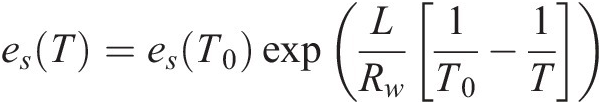where L can be approximated as constant. For vaporization of liquid water, L has a value of 2.50 × 106 J kg–1 at 0 °C and varies with temperature TC (in degrees Celsius) as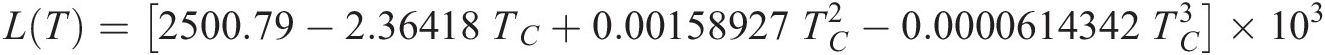For sublimation at 0 °C, L is 2.83 × 106 J kg–1. Latent heat is released to the atmosphere (warming) when clouds condense from the gas phase; conversely, latent heat is absorbed from the atmosphere (cooling) when clouds evaporate.

Figure 2.5 Phase diagram for water describing the stable phases present at equilibrium as a function of water vapor pressure and temperature. The thin line represents the metastable equilibrium between gas and liquid below 0 °C.Reproduced from Jacob

The thin line in the phase diagram of Figure 5 represents the metastable phase equilibrium between water vapor and liquid water at temperatures below 0 °C. This equilibrium is relevant to the atmosphere because of supercooling of liquid clouds. When ice crystals do form in such clouds, the water vapor at equilibrium with the ice is lower than that at equilibrium with the supercooled liquid; thus, the liquid cloud droplets evaporate, transferring their water to the ice crystals. This transfer of water can also take place by collision between the supercooled liquid cloud droplets and the ice crystals (riming). In either case, the resulting rapid growth of the ice crystals promotes precipitation. The heat release associated with the conversion from liquid to ice also adds to the buoyancy of air parcels, fostering further rise and additional condensation and precipitation. Such precipitation formation in mixed-phase clouds is known as the Bergeron process.